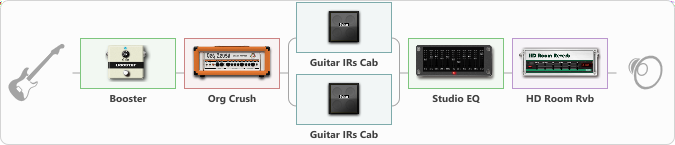# Peavey XXX [live room]

Discussion in 'ToneLib-GFX presets' started by Jean C.Guion, Aug 6, 2020.

1. Peavey XXX [live room]

Preset name: Peavey XXX [live room]

this is the xxx rythme channel ,,,chunky , dynamic and brite//////Use the booster gain to control the amps distortion /gain level////the cabinet output volumes should be used to tune topend/bottom end response...

Effects chain:Effect: "Booster" (Dynamics / Filter), active - "yes"
{
"Gain" = 40
}

Effect: "Org Crush" (Amp simulators), active - "yes"
{
"Gain" = 33
"Bass" = 58
"Middle" = 80
"Treble" = 31
"Presence" = 59
"Master" = 79
"Level (dB)" = -3
}

Effect: "Splitter" (Dynamics / Filter), active - "yes"
{
"A-Bypass" = Off
"A-Pan" = 0
"A-Level" = 60
"B-Bypass" = Off
"B-Pan" = 0
"B-Level" = 60

'A' branch:
{

Effect: "Guitar IRs Cab" (Cabinets), active - "yes"
{
"Model" = Orange PPC (4x12")
"Mic Position" = Middle
"Mic Distance" = Middle
"Low Cut (Hz)" = 79
"Hi Cut (kHz)" = 3.0
"Mix" = 100
"Level (dB)" = 7
}
}
'B' branch:
{

Effect: "Guitar IRs Cab" (Cabinets), active - "yes"
{
"Model" = Fender Deluxe Rev (1x12")
"Mic Position" = Edge
"Mic Distance" = Far
"Low Cut (Hz)" = 135
"Hi Cut (kHz)" = 6.0
"Mix" = 100
"Level (dB)" = 3
}
}
}

Effect: "Studio EQ" (Dynamics / Filter), active - "yes"
{
"31 Hz" = -9
"62 Hz" = 2
"125 Hz" = -3
"250 Hz" = 2
"500 Hz" = 1
"1 kHz" = 0
"2 kHz" = 0
"4 kHz" = -1
"8 kHz" = -3
"16 kHz" = -2
"Level (dB)" = -1
}

Effect: "HD Room Rvb" (Reverberation), active - "yes"
{
"Time" = 5.8
"PreLPF" = 70
"PreDelay" = 104
"HiDamp" = 86
"LoGain" = -4.6
"Mix" = 39
}

Note: You will need to download and install the ToneLib-GFX software to use the preset.

File size:
986 bytes
Views:
2,993